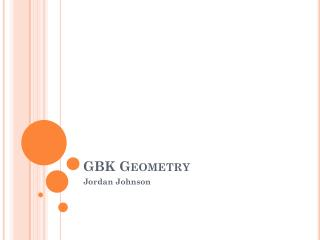# GBK Geometry - PowerPoint PPT PresentationDownload PresentationGBK Geometry

Presentation Description
Download Presentation## GBK Geometry

- - - - - - - - - - - - - - - - - - - - - - - - - - - E N D - - - - - - - - - - - - - - - - - - - - - - - - - - -
##### Presentation Transcript

1. GBK Geometry Jordan Johnson

2. Today’s plan • Greeting • Homework Questions? • (pp. 392-394, review proof, Asgs #67-70) • Practice Quiz • Return work • Lesson & Proof: • Applying the Side-Splitter Theorem • Proving the AA Theorem • Homework / Questions • Clean-up

3. Practice Quiz • Iff a⁄b = b⁄c, then b is the _____________ of a and c. • Find the exact means of... Arithmetic Geometric • 5 and 45 • 14 and 2 • 81 and 9 • Find two pairs of numbers whose geometric mean is 10.

4. How to Build a House Quickly...?

5. A Problem

6. AA Similarity • Theorem 45 (The AA Similarity Theorem): • If two angles of one triangle are equal to two angles of another triangle, then the two triangles are similar. • Given: • Triangles ABC and DEF. • A = D and B = E. • Prove:ABC ~ DEF. • Strategy: Prove C = F, then prove all pairs of sides are proportional.

7. Homework • Log 25+ minutes: • Asgs #67-70 • Proof: • Prove: If ΔABC ΔDEF, then ΔABC ~ΔDEF. • Prove that similarity is transitive. • Prove the AA Similarity Theorem. • Unit 9 Test Analysis (due Wed.)

8. Clean-up / Reminders • Pick up all trash / items. • Push in chairs (at front and back tables). • See you tomorrow!